## Functional components

``````<template>
<div class="cell">
<div v-if="value" class="on"></div>
<section v-else class="off"></section>
</div>
</template>

<script>
export default {
props: ['value'],
}
</script>
``````

``````<template functional>
<div class="cell">
<div v-if="props.value" class="on"></div>
<section v-else class="off"></section>
</div>
</template>
``````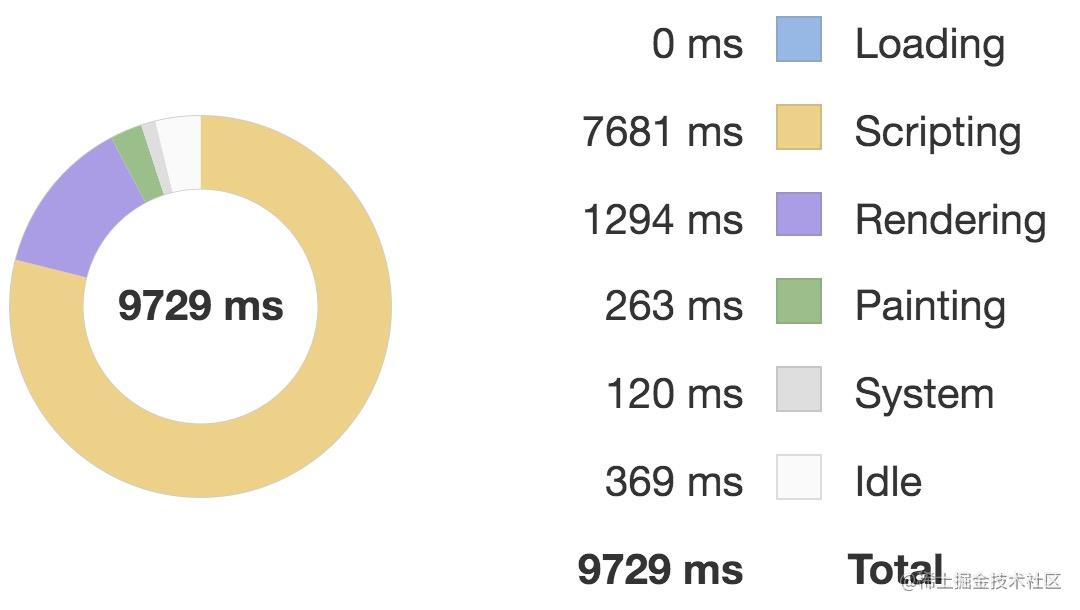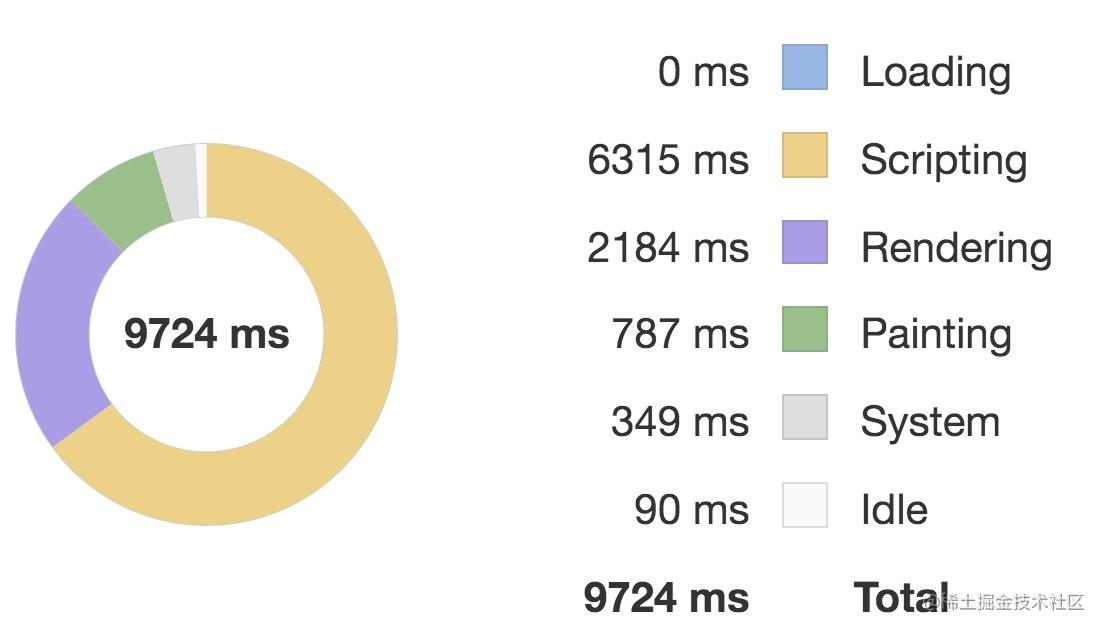## Child component splitting

``````<template>
<div :style="{ opacity: number / 300 }">
<div>{{ heavy() }}</div>
</div>
</template>

<script>
export default {
props: ['number'],
methods: {
heavy () {
const n = 100000
let result = 0
for (let i = 0; i < n; i++) {
result += Math.sqrt(Math.cos(Math.sin(42)))
}
return result
}
}
}
</script>
``````

``````<template>
<div :style="{ opacity: number / 300 }">
<ChildComp/>
</div>
</template>

<script>
export default {
components: {
ChildComp: {
methods: {
heavy () {
const n = 100000
let result = 0
for (let i = 0; i < n; i++) {
result += Math.sqrt(Math.cos(Math.sin(42)))
}
return result
},
},
render (h) {
return h('div', this.heavy())
}
}
},
props: ['number']
}
</script>
``````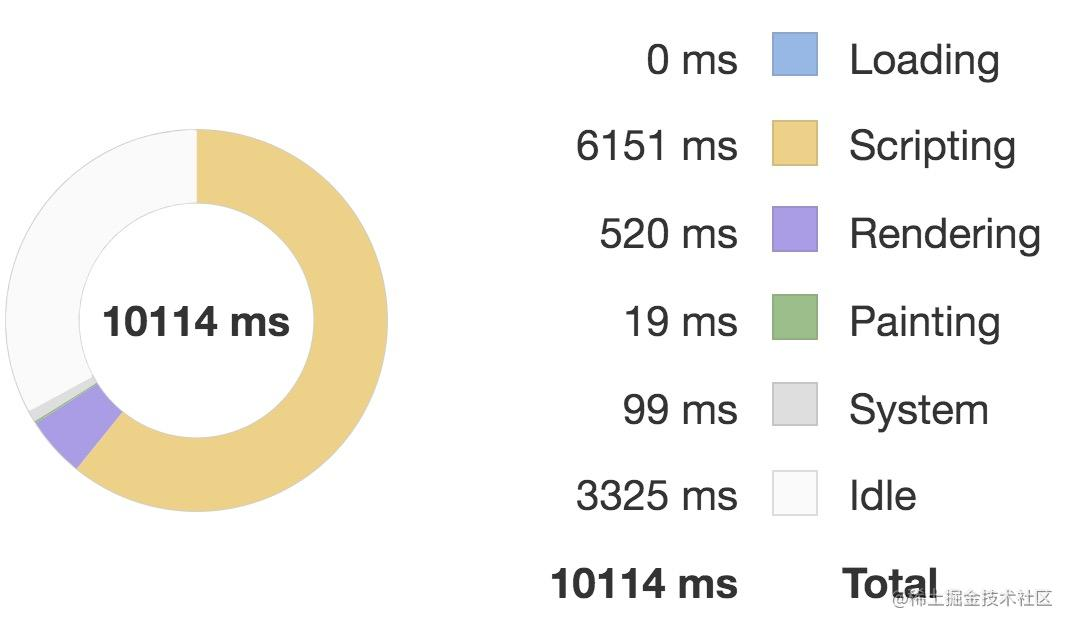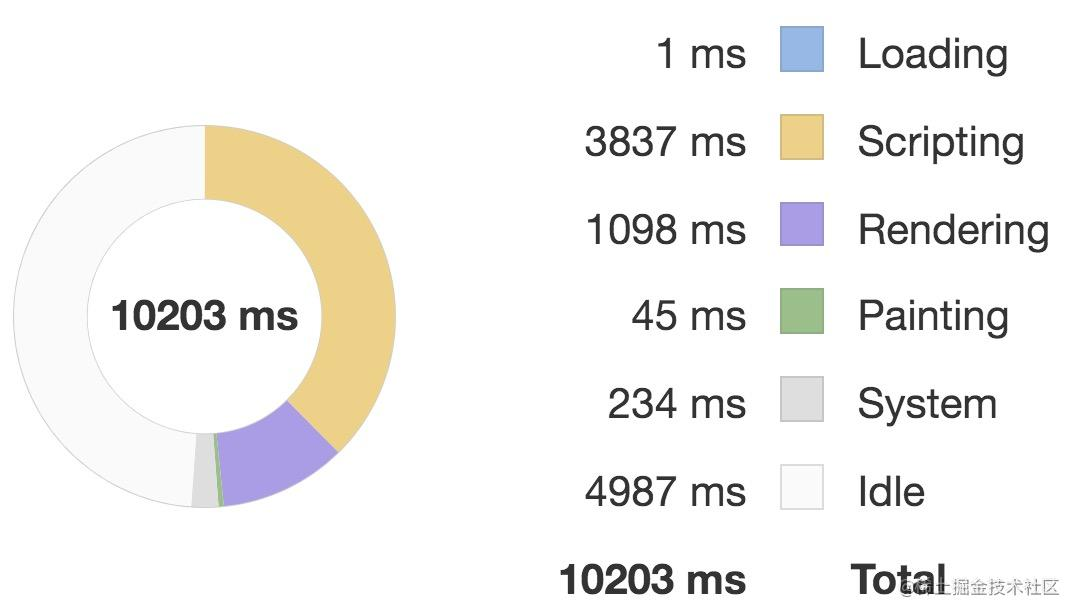## Local variables

``````<template>
<div :style="{ opacity: start / 300 }">{{ result }}</div>
</template>

<script>
export default {
props: ['start'],
computed: {
base () {
return 42
},
result () {
let result = this.start
for (let i = 0; i < 1000; i++) {
result += Math.sqrt(Math.cos(Math.sin(this.base))) + this.base * this.base + this.base + this.base * 2 + this.base * 3
}
return result
},
},
}
</script>
``````

``````<template>
<div :style="{ opacity: start / 300 }">{{ result }}</div>
</template>

<script>
export default {
props: ['start'],
computed: {
base () {
return 42
},
result ({ base, start }) {
let result = start
for (let i = 0; i < 1000; i++) {
result += Math.sqrt(Math.cos(Math.sin(base))) + base * base + base + base * 2 + base * 3
}
return result
},
},
}
</script>
``````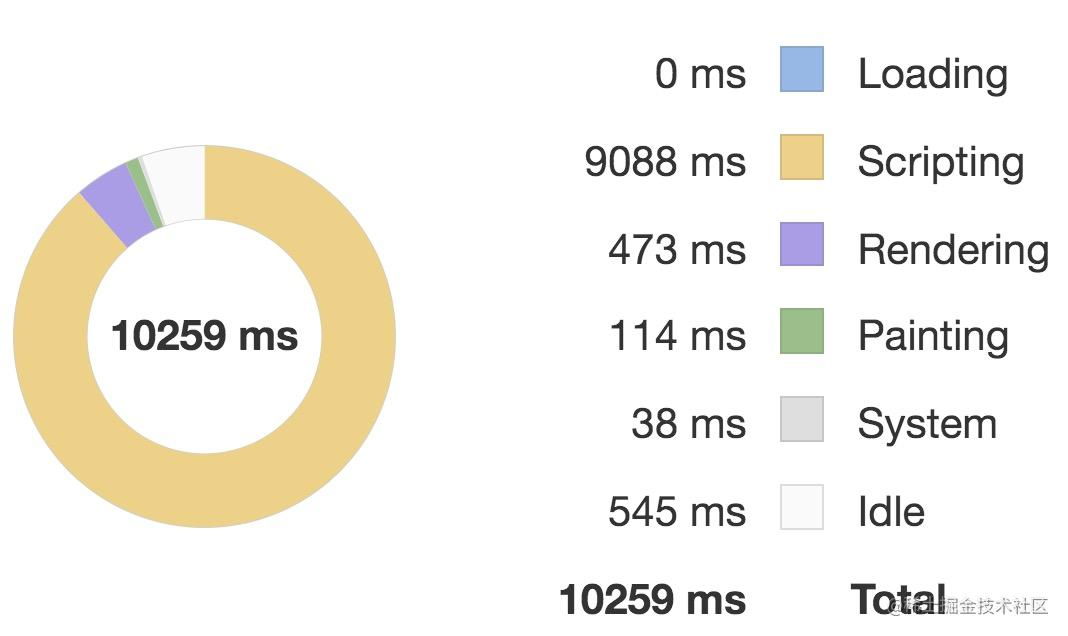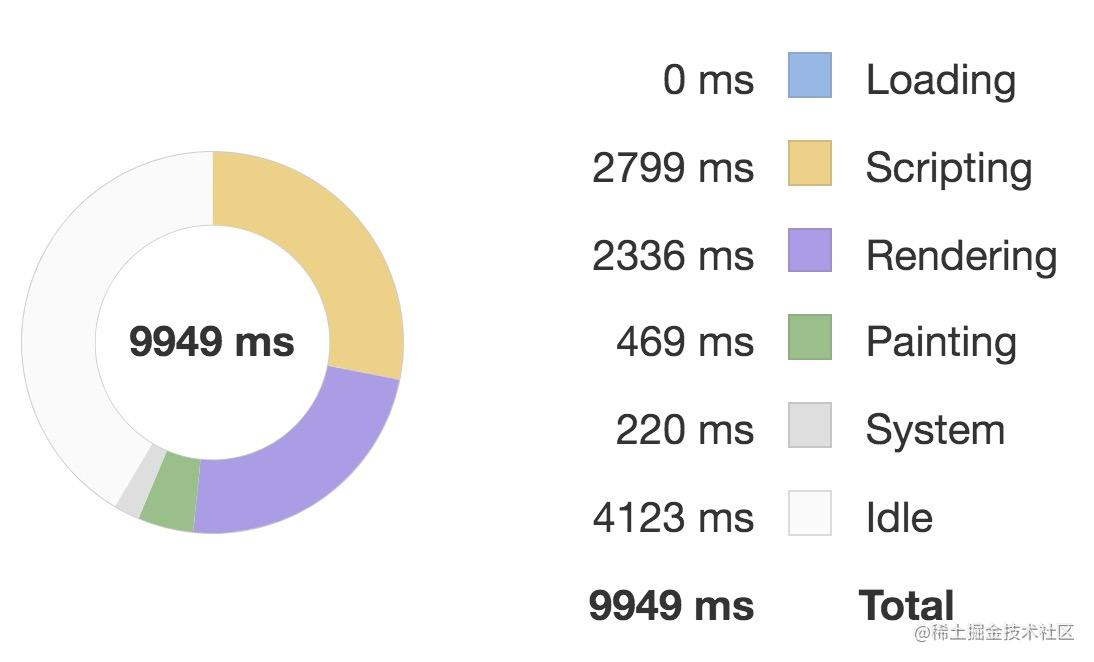## Reuse DOM with v-show

``````<template functional>
<div class="cell">
<div v-if="props.value" class="on">
<Heavy :n="10000"/>
</div>
<section v-else class="off">
<Heavy :n="10000"/>
</section>
</div>
</template>

``````

``````<template functional>
<div class="cell">
<div v-show="props.value" class="on">
<Heavy :n="10000"/>
</div>
<section v-show="!props.value" class="off">
<Heavy :n="10000"/>
</section>
</div>
</template>
``````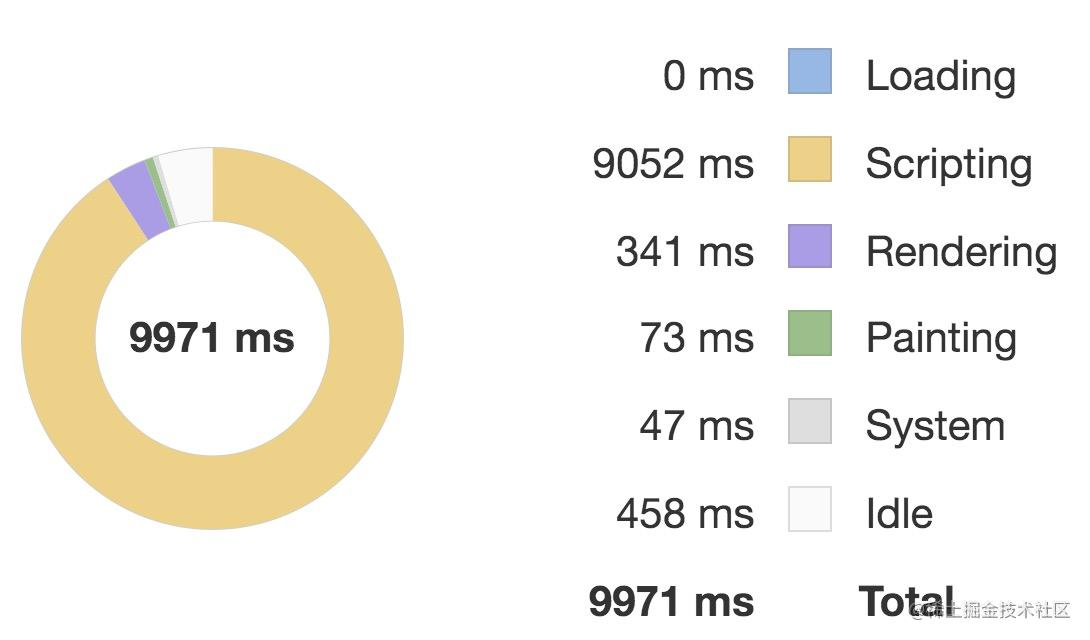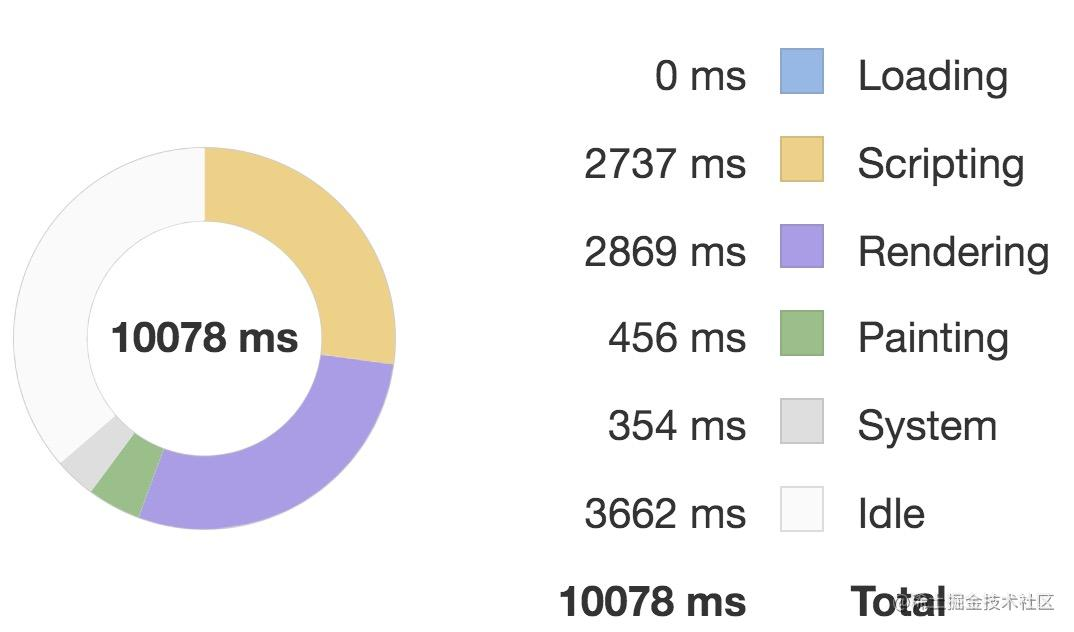`v-if` 指令在编译阶段就会编译成一个三元运算符，条件渲染，比如优化前的组件模板经过编译后生成如下渲染函数：

``````function render() {
with(this) {
return _c('div', {
staticClass: "cell"
}, [(props.value) ? _c('div', {
staticClass: "on"
}, [_c('Heavy', {
attrs: {
"n": 10000
}
})], 1) : _c('section', {
staticClass: "off"
}, [_c('Heavy', {
attrs: {
"n": 10000
}
})], 1)])
}
}
``````

``````function render() {
with(this) {
return _c('div', {
staticClass: "cell"
}, [_c('div', {
directives: [{
name: "show",
rawName: "v-show",
value: (props.value),
expression: "props.value"
}],
staticClass: "on"
}, [_c('Heavy', {
attrs: {
"n": 10000
}
})], 1), _c('section', {
directives: [{
name: "show",
rawName: "v-show",
value: (!props.value),
expression: "!props.value"
}],
staticClass: "off"
}, [_c('Heavy', {
attrs: {
"n": 10000
}
})], 1)])
}
}
``````

## KeepAlive

``````<template>
<div id="app">
<router-view/>
</div>
</template>
``````

``````<template>
<div id="app">
<keep-alive>
<router-view/>
</keep-alive>
</div>
</template>
``````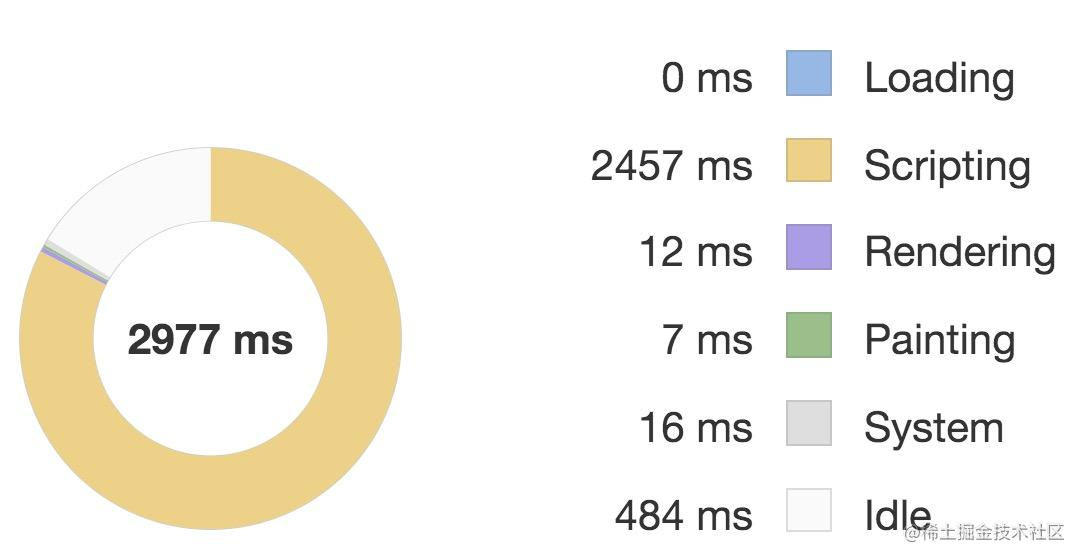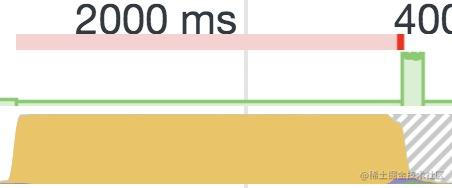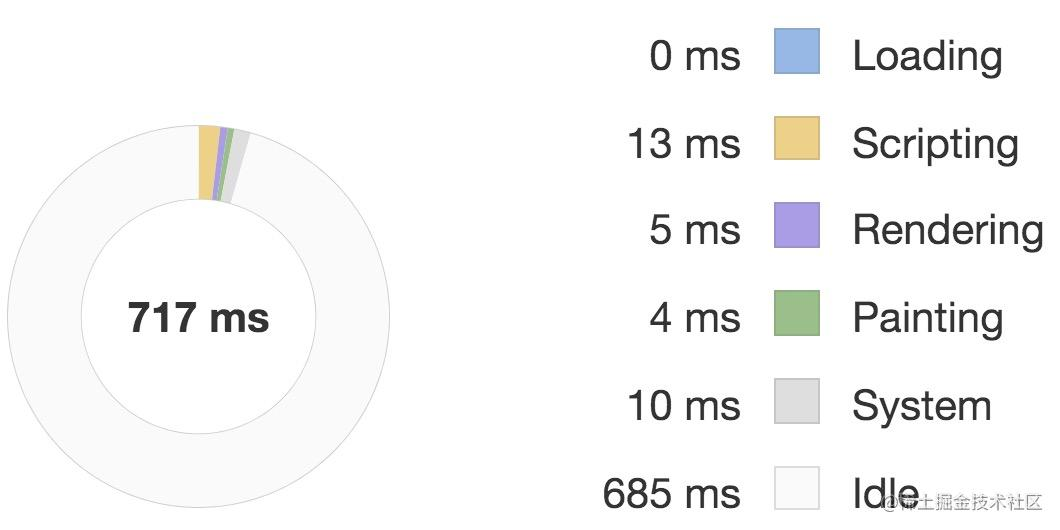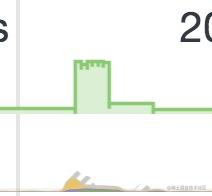## Deferred features

``````<template>
<div class="deferred-off">
<VueIcon icon="fitness_center" class="gigantic"/>

<h2>I'm an heavy page</h2>

<Heavy v-for="n in 8" :key="n"/>

<Heavy class="super-heavy" :n="9999999"/>
</div>
</template>
``````

``````<template>
<div class="deferred-on">
<VueIcon icon="fitness_center" class="gigantic"/>

<h2>I'm an heavy page</h2>

<template v-if="defer(2)">
<Heavy v-for="n in 8" :key="n"/>
</template>

<Heavy v-if="defer(3)" class="super-heavy" :n="9999999"/>
</div>
</template>

<script>
import Defer from '@/mixins/Defer'

export default {
mixins: [
Defer(),
],
}
</script>
``````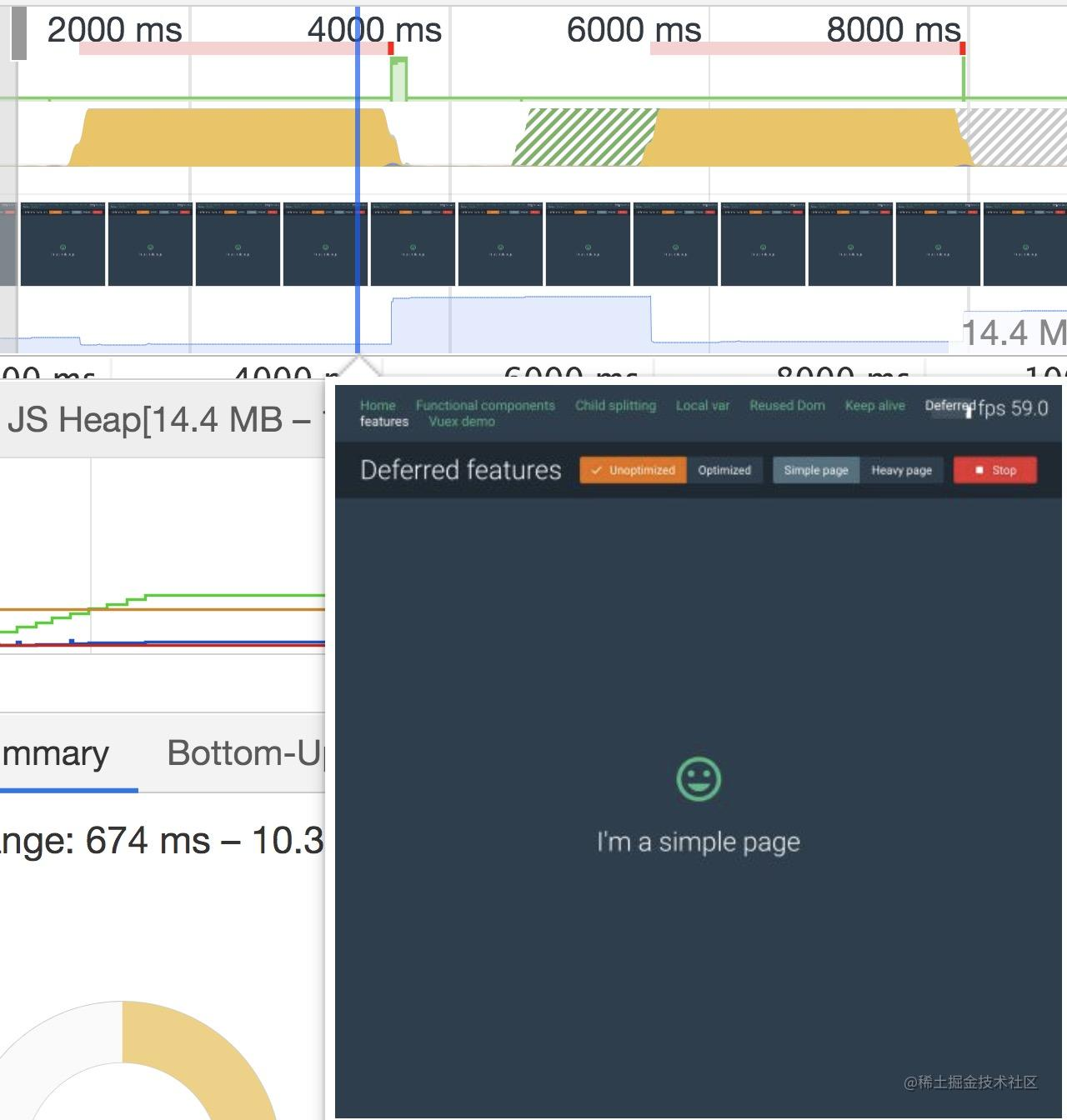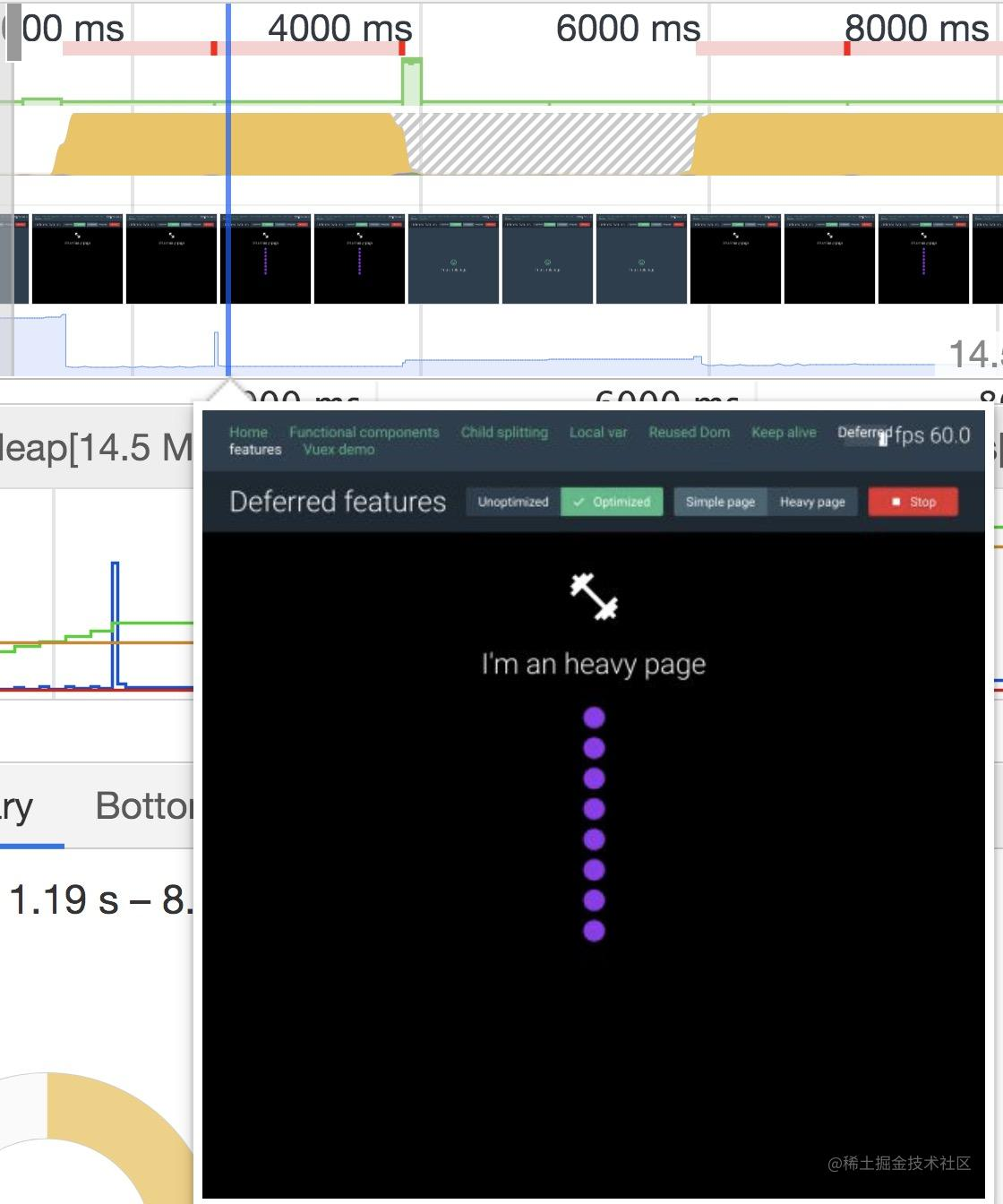``````export default function (count = 10) {
return {
data () {
return {
displayPriority: 0
}
},

mounted () {
this.runDisplayPriority()
},

methods: {
runDisplayPriority () {
const step = () => {
requestAnimationFrame(() => {
this.displayPriority++
if (this.displayPriority < count) {
step()
}
})
}
step()
},

defer (priority) {
return this.displayPriority >= priority
}
}
}
}
``````

`Defer` 的主要思想就是把一个组件的一次渲染拆成多次，它内部维护了 `displayPriority` 变量，然后在通过 `requestAnimationFrame` 在每一帧渲染的时候自增，最多加到 `count`。然后使用 `Defer mixin` 的组件内部就可以通过 `v-if="defer(xxx)"` 的方式来控制在 `displayPriority` 增加到 `xxx` 的时候渲染某些区块了。

## Time slicing

``````fetchItems ({ commit }, { items }) {
commit('clearItems')
}
``````

``````fetchItems ({ commit }, { items, splitCount }) {
commit('clearItems')
const queue = new JobQueue()
splitArray(items, splitCount).forEach(
// 分时间片提交数据
requestAnimationFrame(() => {
done()
})
})
)
await queue.start()
}
``````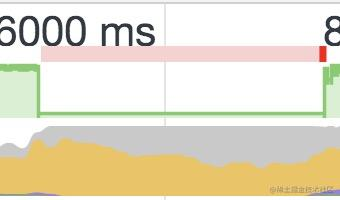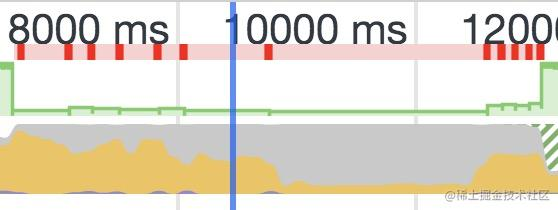## Non-reactive data

``````const data = items.map(
item => ({
id: uid++,
data: item,
vote: 0
})
)
``````

``````const data = items.map(
item => optimizeItem(item)
)

function optimizeItem (item) {
const itemData = {
id: uid++,
vote: 0
}
Object.defineProperty(itemData, 'data', {
// Mark as non-reactive
configurable: false,
value: item
})
return itemData
}
``````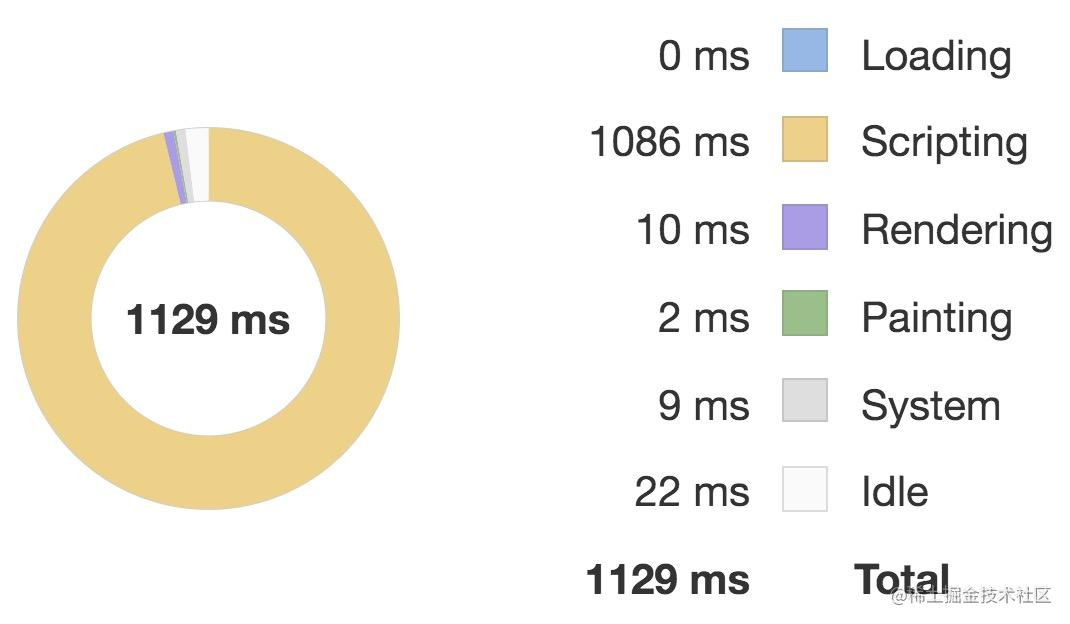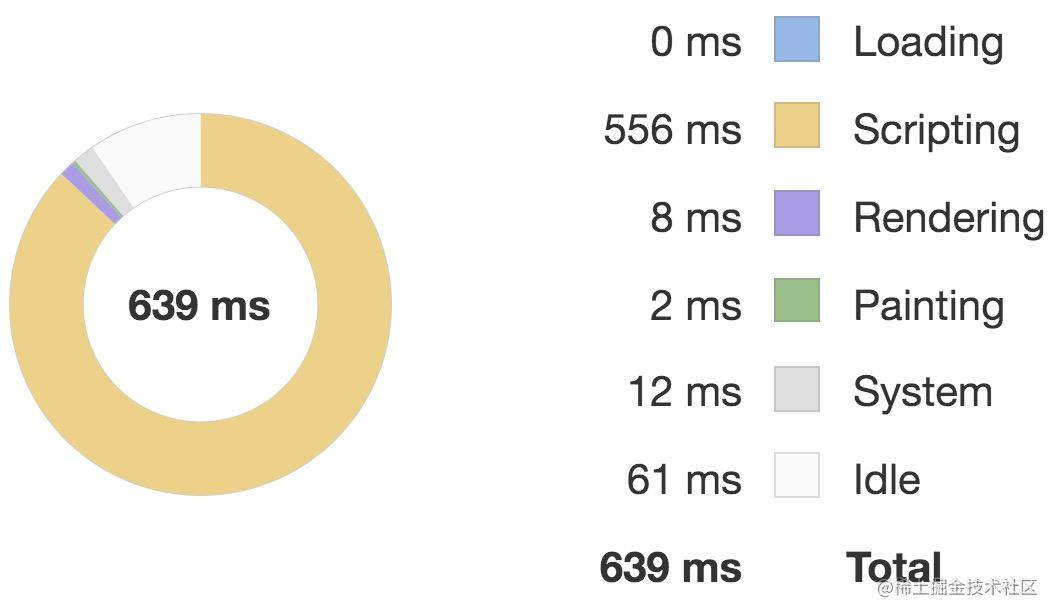``````export default {
created() {
this.scroll = null
},
mounted() {
this.scroll = new BScroll(this.\$el)
}
}
``````

## Virtual scrolling

``````<div class="items no-v">
<FetchItemViewFunctional
v-for="item of items"
:key="item.id"
:item="item"
@vote="voteItem(item)"
/>
</div>
``````

``````<recycle-scroller
class="items"
:items="items"
:item-size="24"
>
<template v-slot="{ item }">
<FetchItemView
:item="item"
@vote="voteItem(item)"
/>
</template>
</recycle-scroller>
``````